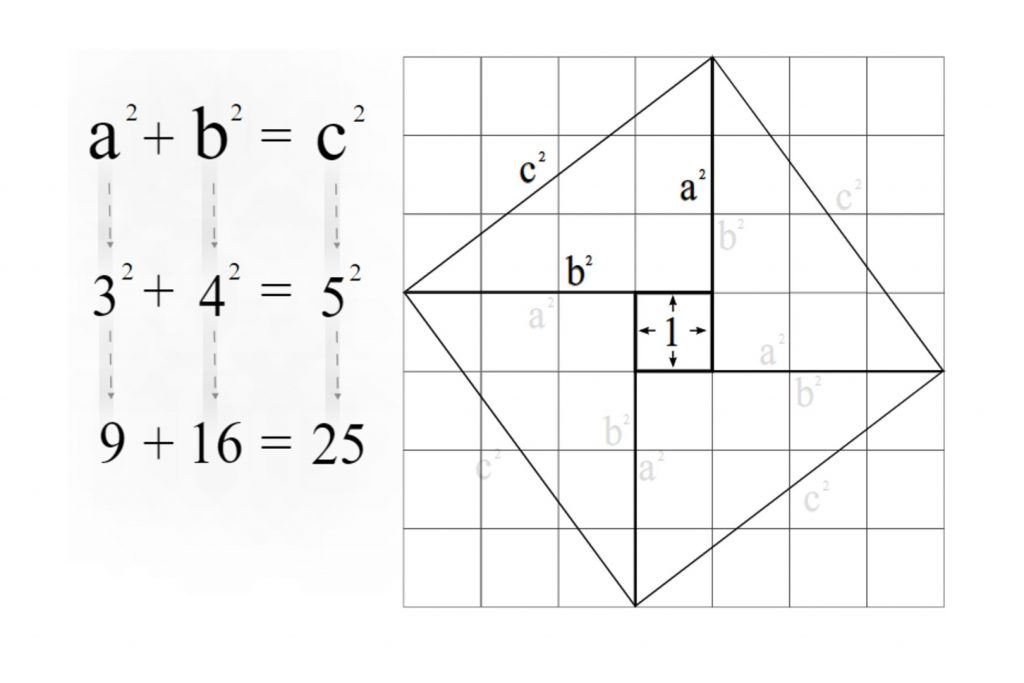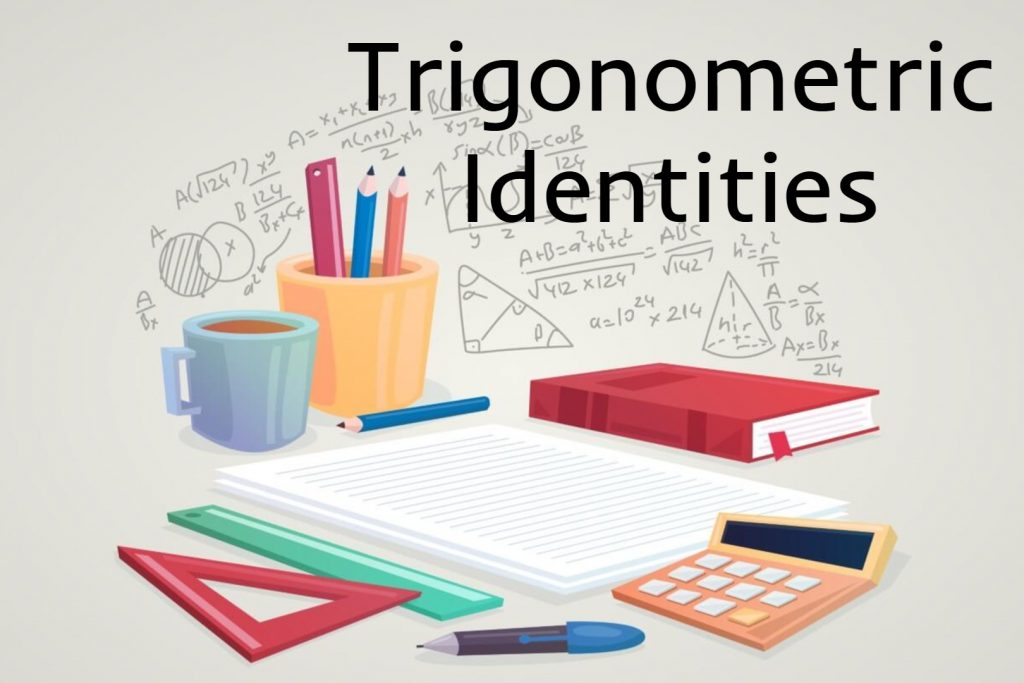# Trigonometric Identities## The Pythagorean Theorem

Right-angled triangle and the Pythagorean theorem In this article lets try to develop the Pythagorean theorem by means of the right angle. So first let’s get some proper ideas about the right-angled triangle. What is a right-angled triangle? If an angle of a triangle is 90o it is called a right-angled triangle or right triangle. …## Trigonometric Identities

A Trigonometric identity or trig identity is an identity that contains the trigonometric functions sine(sin), cosine(cos), tangent(tan), cotangent(cot), secant(sec), or cosecant(csc). Trigonometric identities can use to: Simplify trigonometric expressions. Solve trigonometric equations. Prove that one trigonometric expression is equivalent to another so that we can replace the first expression by the second expression. The following …